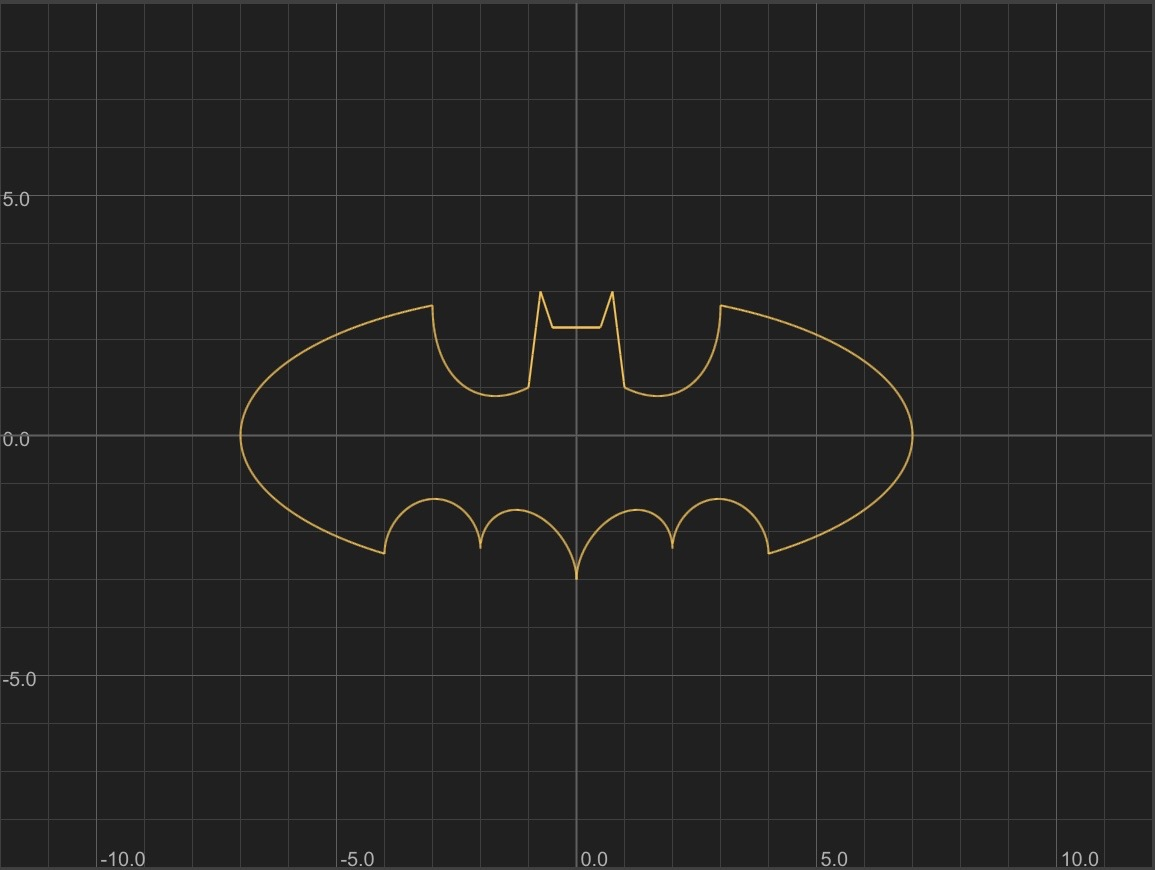HomePosted on 8/7/2021
Tags: Math
With FormulaGraph now available, it's easier than ever to explore more math shapes.

An interesting one is the "Batman Curve".

A professor named J. Matthew Register created this formula to make math fun. Here's what he says about the history in a post on Quora:
"I wrote it many, many years ago. I was teaching at a few art schools throughout the greater Sacramento area, and I used it to engage my students in the topic of graphing. One of my coolest students (Mr. Wilkinson aka i_luv_ur_mom) posted it to Reddit back in 2011 and it went viral. I'm a full time professor over at American River College, doing every thing I can to make math as enjoyable as possible."

There's a nice breakdown of how the formula's separate pieces come together to make the full shape on Math StackExchange.

While the 6-formula version graphs ok (see here or on Desmos), I noticed that the original single formula version does not work in FormulaGraph or Desmos (it forms a blank graph showing no solutions). User copper.hat on Math StackExchange notes why: there are several terms which introduce an imaginary number (by taking sqrt of a negative number). For this reason, the combined formula does not have the solutions required for the necessary range of x and y values. (Wolfram also has a writeup that notes that the Batman Curve is defined piecewise.)

The original formula uses the trick of undefined/imaginary values to truncate shapes to a specific range of x values. Since this trick breaks the formula, I wondered if it's possible to find a different gadget that could constrain x values without introducing undefined/imaginary values.

Here's what I came up with:
For a formula F, constrain its x values to the interval [L, U] with:

((F)^2 + min(1, ceil(abs(min(0, U-x)))) + min(1, ceil(abs(max(0, L-x))))) = 0
To fix the Batman Curve, I went segment-by-segment applying the gadget. First, I needed to resolve any undefined/imaginary results. Second, I needed to apply my gadget for the relevant x (and sometimes y) ranges.

Here's the crazy formula I ended up with:
(((2.25-y)^2+min(1, ceil(abs(min(0, 0.5-x))))+min(1, ceil(abs(max(0, -0.5-x))))) * ((abs(x/2)-((3*sqrt(33)-7)/112)*(x^2)-3+sqrt(abs(1-(abs(abs(x)-2)-1)^2))-y)^2 + min(1, ceil(abs(min(0, 4-x))))+min(1, ceil(abs(max(0, -4-x)))))) * (((min(1, ceil(abs(max(0, 3-x))))+min(1, ceil(abs(min(0, 7-x))))) + min(1, ceil(abs(max(0, 0-y))))+ (((x/7)^2)+((y/3)^2)-1)) * ((min(1, ceil(abs(max(0, 4-x))))+min(1, ceil(abs(min(0, 7-x))))) + min(1, ceil(abs(min(0, 0-y))))+ (((x/7)^2)+((y/3)^2)-1)) * ((min(1, ceil(abs(max(0, 3-(-x)))))+min(1, ceil(abs(min(0, 7-(-x)))))) + min(1, ceil(abs(max(0, 0-y))))+ (((-x/7)^2)+((y/3)^2)-1)) * ((min(1, ceil(abs(max(0, 4-(-x)))))+min(1, ceil(abs(min(0, 7-(-x)))))) + min(1, ceil(abs(min(0, 0-y))))+ (((-x/7)^2)+((y/3)^2)-1)) + min(1, ceil(abs(max(0, 1-abs(x)))))) * ((min(1, ceil(abs(min(0, 3-x))))+min(1, ceil(abs(max(0, 1-x))))) + ((6*sqrt(10)/7+(1.5-0.5*abs(x)) -6*sqrt(10)/14*sqrt(abs(4-(abs(x)-1)^2))-y))^2) * ((min(1, ceil(abs(min(0, 3+x))))+min(1, ceil(abs(max(0, 1+x))))) + ((6*sqrt(10)/7+(1.5-0.5*abs(-x)) -6*sqrt(10)/14*sqrt(abs(4-(abs(-x)-1)^2))-y))^2) * ((min(1, ceil(abs(max(0, 1-y))))+min(1, ceil(abs(min(0, 3-y))))) + (9-8*abs(x)-y)^2) * ((min(1, ceil(abs(max(0, 2.25-y))))+min(1, ceil(abs(min(0, 3-y))))) + (3*abs(x)+0.75-y)^2) = 0

This curve is pictured at the top of this post!

(See my raw stream of consciousness notes as I went step-by-step)

There are a few more Batman Curves that I found on the pages linked above:

Here's a different and simpler formula (though I don't think it looks as good) from copper.hat's post on Math StackExchange:
0 = -1 +
(1/36)*x^2+(1/9)*y^2 +
max(0, 2-(1.5*(x+3)^2+1*(y+2.7)^2)) +
max(0, 2-(1.5*(x-3)^2+1*(y+2.7)^2)) +
max(0, 2 - (1.9*((5*(x+1)+(y+3.5))/sqrt(26))^2 + 1/1.7 * ((-(x+1)+5*(y+3.5))/sqrt(26)))^2)) -
max(0, 2 - (1.9*((5*(x-1)-(y+3.5))/sqrt(26))^2 + (1/1.7) * (((x-1)+5*(y+3.5))/sqrt(26)))^2) -
max(0,2-((1.1*(x-2))^4-(y-2.1))) -
max(0,2-((1.1*(x+2))^4-(y-2.1))) +
max(0,2-((1.5*x)^8-(y-3.5)))

J. Matthew Register shared a newer formula on his Quora post:
(3*sqrt(4-(abs(x)-2)^2)+abs(x)-20-4*y)
*
(3*sqrt(4-(abs(x)-6)^2)+abs(x)-20-4*y)
*
(x^2+4*(y^2)-100*sqrt(abs((7-abs(2*y-1)))/(7-abs(2*y-1))))
*
(2*((abs(x)-3)^2)-9*y+18*sqrt(abs((2-abs((abs(x)-4))))/(2-abs((abs(x)-4)))))
*
(-68*abs((abs(x)-3/2))-9*y+54*sqrt(abs((43-abs((136*abs(x)-229))))/(43-abs((136*abs(x)-229)))))
*
(y-5*sqrt(abs((1-abs(x)))/(1-abs(x)))) = 0